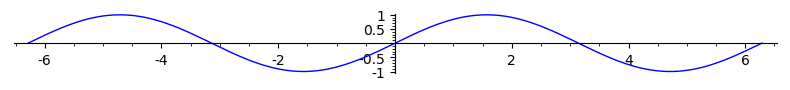# Revision history [back]

You may do:

sage: p = plot(sin(x), (x, -2*pi, 2*pi))
sage: p.show(figsize=[8,1])


to change the scaling:The method save accepts the same argument:

sage: p.save('image.png', figsize=[8,1])


To do further changes on the figure, you may get the matplotlib object behind the 2d Graphics object and use matplotlib possibilities:

sage: figure = p.matplotlib()
sage: figure
<Figure size 640x480 with 1 Axes>
sage: axes = figure.axes


You may do:

sage: p = plot(sin(x), (x, -2*pi, 2*pi))
sage: p.show(figsize=[8,1])


to change the scaling:The method save accepts the same argument:

sage: p.save('image.png', figsize=[8,1])


To do further changes on the figure, you may get the matplotlib object behind the 2d Graphics object and use matplotlib possibilities:

sage: figure = p.matplotlib()
sage: figure
<Figure size 640x480 with 1 Axes>
sage: type(figure)
<class 'matplotlib.figure.Figure'>
sage: axes = figure.axes


You may do:

sage: p = plot(sin(x), (x, -2*pi, 2*pi))
sage: p.show(figsize=[8,1])


to change the scaling:The method save accepts the same argument:

sage: p.save('image.png', figsize=[8,1])


To do further changes on the figure, you may get the matplotlib object behind the 2d Graphics object and use matplotlib possibilities:

sage: figure = p.matplotlib()
p.matplotlib(figsize=[8,6])
sage: figure
<Figure size 640x480 800x600 with 1 Axes>
sage: type(figure)
<class 'matplotlib.figure.Figure'>
sage: axes = figure.axes